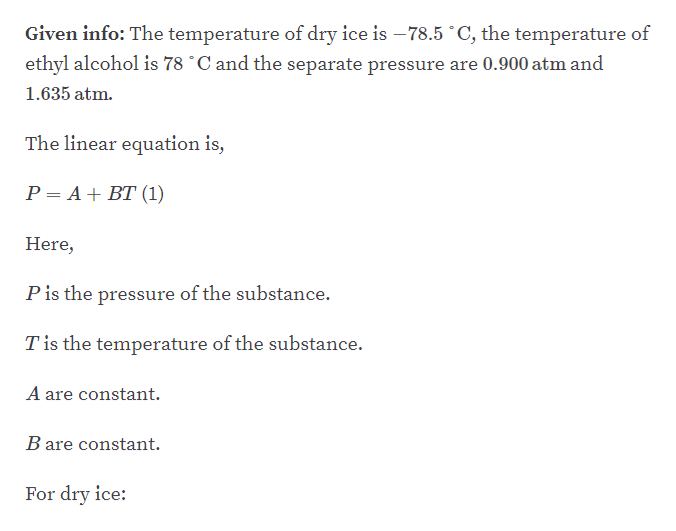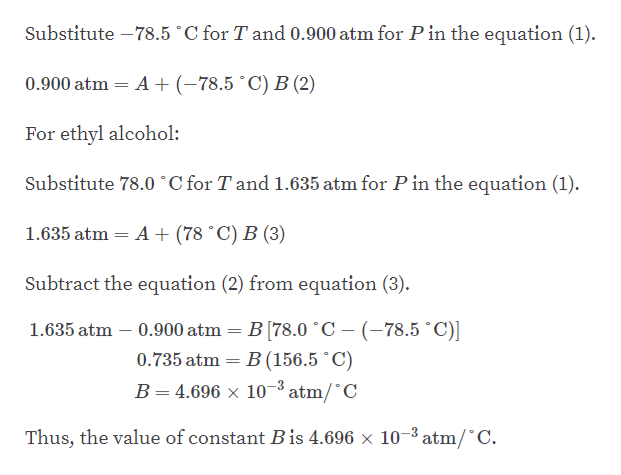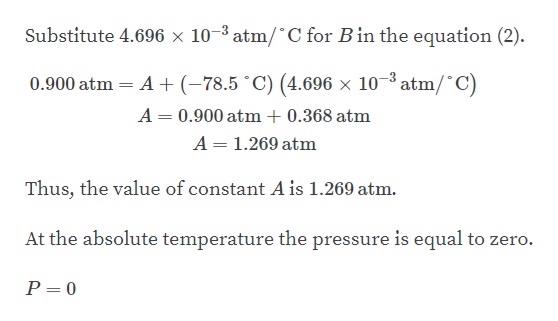# In a student experiment, a constant-volume gas thermometer is calibrated in dry ice (-78.5°C) and in boiling ethyl alcohol (78.0°C). The separate pressures are 0.900 atm and 1.635 atm. (a) What value of absolute zero in degrees Celsius does the calibration yield? What pressures would be found at (b) the freezing and (c) boiling points of water? Hint : Use the linear relationship P = A + BT, where A and B are constants.

Question
1 views

In a student experiment, a constant-volume gas thermometer is calibrated in dry ice (-78.5°C) and in boiling ethyl alcohol (78.0°C). The separate pressures are 0.900 atm and 1.635 atm. (a) What value of absolute zero in degrees Celsius does the calibration yield? What pressures would be found at (b) the freezing and (c) boiling points of water? Hint : Use the linear relationship P = A + BT, where A and B are constants.

check_circle

Step 1

Part A:help_outlineImage TranscriptioncloseGiven info: The temperature of dry ice is – 78.5 °C, the temperature of ethyl alcohol is 78 °C and the separate pressure are 0.900 atm and 1.635 atm. The linear equation is, P= A+ BT (1) Here, Pis the pressure of the substance. Tis the temperature of the substance. A are constant. B are constant. For dry ice: fullscreen
Step 2help_outlineImage TranscriptioncloseSubstitute –78.5 °C for T and 0.900 atm for P in the equation (1). 0.900 atm = A+ (-78.5 °C) B (2) For ethyl alcohol: Substitute 78.0 °C for T and 1.635 atm for P in the equation (1). 1.635 atm = A + (78 °C) B (3) Subtract the equation (2) from equation (3). 1.635 atm – 0.900 atm = B [78.0 °C – (-78.5 °C)] 0.735 atm = B (156.5 °C) B= 4.696 × 10-³ atm/°C Thus, the value of constant B is 4.696 × 10-3 atm/°C. fullscreen
Step 3help_outlineImage TranscriptioncloseSubstitute 4.696 × 10-3 atm/°C for B in the equation (2). 0.900 atm = A +(-78,5 °C) (4.696 × 10-³ atm/°C) A = 0.900 atm + 0.368 atm A = 1.269 atm Thus, the value of constant A is 1.269 atm. At the absolute temperature the pressure is equal to zero. P = 0 fullscreen

### Want to see the full answer?

See Solution

#### Want to see this answer and more?

Solutions are written by subject experts who are available 24/7. Questions are typically answered within 1 hour.*

See Solution
*Response times may vary by subject and question.
Tagged in

### Physics